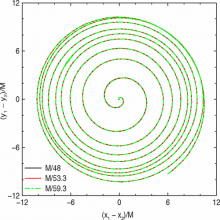Comparison of Numerical and Post-Newtonian Waveforms for Generic Precessing Black-Hole Binaries
By Manuela Campanelli, Carlos O. Lousto, Hiroyuki Nakano, Yosef Zlochower
Published in Physical Review D 79, 084010 (Sunday, April 5, 2009)

## Abstract

We compare the waveforms and orbital dynamics from the first long-term, fully non-linear, numerical simulations of generic black-hole binaries with post-Newtonian predictions. Our binaries have mass ratios q~0.8 and arbitrarily oriented spins with magnitudes S_1/m_12~0.6 and S_2/m_22~0.4 and orbit 9 times prior to merger. The binaries start at an initial orbital separation of r~11M, with orbital parameters determined by 2.5PN and 3.5PN post-Newtonian evolutions of a quasi-circular binary that started at r=50M. The resulting binaries have essentially no eccentricity according to the 2.5PN and 3.5PN systems, but show significant eccentricity in the numerical simulations; although 3.5PN significantly reduces the eccentricity of the binary compared to 2.5PN. We perform three numerical evolutions from r~11M with maximum resolutions of h=M/48,M/53.3,M/59.3, to verify numerical convergence. We observe a reasonably good agreement between the PN and numerical waveforms, with an overlap of nearly 99% for the first six cycles of the (l=2,m=+-2) modes, 94% for the (l=2,m=+-1) modes, and nearly 91% for the (l=3,m=+-3) modes. The phase differences between numerical and post-Newtonian approximations appear to be independent of the (l,m) modes considered and relatively small for the first 3-4 orbits. A clear advantage of the 3.5 PN model over the 2.5 PN one is observed. In addition, we identify features in the waveforms related to precession.

## CCRG Authors

Campanelli, Manuela

## Research Areas

Analytic Relativity & Modeling